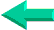# VBA Lesson 16: Cells, Ranges, Columns and Rows in VBA for Excel

A lot of VBA beginners start their career using Cells. For example:
Cells(1,1).Select is the same thing as Range("A1").Select and
Cells(11,31).Select
is the same as Range("AE11").Select.

We strongly recommend that you use Range instead of Cells to work with cells and groups of cells. It makes your sentences much clearer and you are not forced to remember that column AE is column 31.

The only time that you will use Cells is when you want to select all the cells of a worksheet. For example:
Cells.Select
To select all cells and then to empty all cells  of values or formulas  you will use:
Cells.ClearContents

## Range

To select a single cell you will write:
Range
("A1").Select

To select a set of contiguous cells you will use the colon and write:
Range("A1:G5").Select

To select a set of non contiguous cells you will use the comma and write:
Range("A1,A5,B4").Select

To select a set of non contiguous cells and a range you will use both the colon and the comma:
Range("A1,A5,B4:B8").Select

## Offset

The Offset property is the one that you will use the most with Range to move around the sheet.

To move one cell down (from B2 to B3): Range("B2").Offset(1,0).Select
To move one cell to the right (from B2 to C2): Range("B2").Offset(0,1).Select
To move one cell up  (from B2 to B1): Range("B2").Offset(-1,0).Select
To move one cell to the left  (from B2 to A2): Range("B2").Offset(0,-1).Select

To move one cell down from the selected cell:
ActiveCell.Offset(1,0).Select

As you notice the first argument between the parentheses for Offset is the number of rows and the second one is the number of columns. So to move from A1 to G6 you will need:
Range("A1").Offset(5,6).Select

You will use very often the following piece of code . It selects a cell and 4 more to the right to be copied/pasted somewhere else:
Range(ActiveCell,ActiveCell.Offset(0,4)).Copy
Notice the comma after the first
ActiveCell and the double closing parentheses before the Select.

Go to the next lesson
Lesson
17 : Message and Input Boxes (MsgBox, InputBox) in ExcelBack home